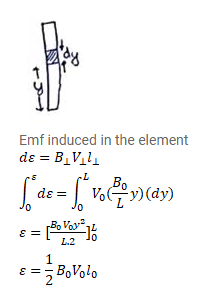# The magnetic field in a region is given by

Question:

The magnetic field in a region is given by $\vec{B}=\vec{k} \frac{B_{0}}{L} y$ where $\mathrm{L}$ is a fixed length. A conducting rod of length $\mathrm{L}$ lies along the $Y$ axis between the origin and the point $(0, L, 0)$. If the rod moves with a velocity $v=v_{0} \bar{l}$, find the emf induced between the ends of the rod.

Solution: